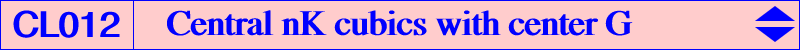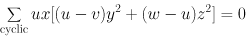All central nK cubics centered at G are nK0++ cubics and have their pole W and their root R at infinity. If R = (u : v : w) is a point at infinity, then the corresponding pole is W = (v - w : w - u : u - v) which is a point on the curve. Note that R and W are the infinite points of two conjugated diameters with respect to the Steiner ellipse. These cubics always have three real concurring asymptotes at G : one is the line GW, the two other are the parallels at G to the asymptotes of the hyperbola (H) isoconjugate of the line at infinity. (H) passes through G and its center is the barycentric square of W. It is the antipode on the Steiner inscribed ellipse of the contact with this ellipse of the trilinear polar of R i.e. the point (u^2 : v^2 : w^2), barycentric square of R. The inflexional tangent at G to the nK passes through the center of this hyperbola. (H) is a rectangular hyperbola if and only if R = X(524), infinite point of the line GK. This hyperbola is the Kiepert hyperbola and the corresponding central cubic is K068. See also K398, K399 and the two Steiner Central nK0++ cubics K400a and K400b. See Special isocubics §3.3 and a generalization in the page P-conical cubics. The general equation is :